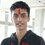# Clarification about the problem "Danger? I laugh at danger!"

In this problem, when we consider rotation about the IAR, then it becomes as follows -I1I2

\begin{aligned} \mathrm{d}B &=\dfrac{\mu_o}{4\pi} \dfrac{\mathrm{d}q}{\mathrm{d}t} \dfrac{\vec{\mathrm{d}l} \times \vec{a}}{|\vec{a}|^3}\\ &=\dfrac{\mu_o}{4\pi}\mathrm{d}q \dfrac{2vr\sin(\theta/2) d}{(r^2+d^2)^{3/2}} \end{aligned}

Because $\left|\vec{\left(\dfrac{\mathrm{d}l}{\mathrm{d}t}\right)}\right|=2v\sin (\theta/2)$ and $\left|\vec{\left(\dfrac{\mathrm{d}l}{\mathrm{d}t}\right)} \times \vec{a}\right| = d$

Now, $\mathrm{d}Q=\dfrac{Q}{2\pi}\mathrm{d}\theta$.

So, \begin{aligned} B &=\dfrac{\mu_o}{4\pi^2} \dfrac{Qvd}{(r^2+d^2)^{3/2}} \int_{0}^{2\pi} \sin (\theta/2) \mathrm{d}\theta\\ &=\boxed{\dfrac{\mu_o Qvd}{\pi^2 (r^2+d^2)^{3/2}}} \end{aligned}Note by Pratik Shastri
6 years, 9 months ago

This discussion board is a place to discuss our Daily Challenges and the math and science related to those challenges. Explanations are more than just a solution — they should explain the steps and thinking strategies that you used to obtain the solution. Comments should further the discussion of math and science.

When posting on Brilliant:

• Use the emojis to react to an explanation, whether you're congratulating a job well done , or just really confused .
• Ask specific questions about the challenge or the steps in somebody's explanation. Well-posed questions can add a lot to the discussion, but posting "I don't understand!" doesn't help anyone.
• Try to contribute something new to the discussion, whether it is an extension, generalization or other idea related to the challenge.

MarkdownAppears as
*italics* or _italics_ italics
**bold** or __bold__ bold
- bulleted- list
• bulleted
• list
1. numbered2. list
1. numbered
2. list
Note: you must add a full line of space before and after lists for them to show up correctly
paragraph 1paragraph 2

paragraph 1

paragraph 2

[example link](https://brilliant.org)example link
> This is a quote
This is a quote
    # I indented these lines
# 4 spaces, and now they show
# up as a code block.

print "hello world"
# I indented these lines
# 4 spaces, and now they show
# up as a code block.

print "hello world"
MathAppears as
Remember to wrap math in $$ ... $$ or $ ... $ to ensure proper formatting.
2 \times 3 $2 \times 3$
2^{34} $2^{34}$
a_{i-1} $a_{i-1}$
\frac{2}{3} $\frac{2}{3}$
\sqrt{2} $\sqrt{2}$
\sum_{i=1}^3 $\sum_{i=1}^3$
\sin \theta $\sin \theta$
\boxed{123} $\boxed{123}$

Sort by:

Hi Pratik,

Can you explain where the $\sin\theta/2$ originates in your derivation? On the left hand side you seem to have a scalar $B$ field (one component), while on the right you have a vector expression. When I calculate the components, I have some that cancel when integrated around the circle, and a $z$-component that survives. The $z$-component of the cross product gives $\left(\vec{a}\times d\vec{l}\right)_z = r^2d\theta$.

Staff - 6 years, 9 months ago

$\dfrac{\mathrm{d}l}{\mathrm{d}t}$ is the velocity of the infinitesimally small element $\mathrm{d}\theta$. The distance of this element from the instantaneous axis of rotation can be found using simple geometry. It comes out to be $2r\sin (\theta/2)$. Also, $\vec{\mathrm{d}l}\times \vec{a}$ is simply $d$ as $\vec{\mathrm{dl}}$ is always in the plane of the ring.

- 6 years, 9 months ago

Yes, I found my mistake, I didn't check the $x-y$ components.. but still why will do they cancel out? The top part of the wheel moves faster than the bottom part, right?

- 6 years, 9 months ago

Are you thinking in the reference frame of the wheel or the frame of the ground?

Staff - 6 years, 9 months ago

The ground.

- 6 years, 9 months ago